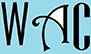## Addition & Subtraction of Fractions: Math Games for Class and Play

Purpose: To make you knowledgeable about  factors and know how to calculate them quickly.

A teacher or an knowledgeable adult may make students form a circle and ask them questions in turns, and whoever gets the answer wrong leaves the circle.  The winner is the last student in the circle.

A teacher or an knowledgeable adult may make students form two or more teams of equal members and ask questions in turns.  When a team gets it right, they get 2 points, but if they get it wrong, they lose 1 point.  If Team A gets it wrong, they lose a point and Team B gets a chance to get 1 point.  If Team B misses the opportunity, they do not lose 1 point because it was not their question originally, then Team C gets the chance to gain the extra point.  The teacher or adult can then answer the question with explanations if all the teams missed it.  Team A loses 1 point, no other team loses a point, and Team B gets the next question.

Game Items: Pencils, pens, and papers are allowed, but each question must be answered within 5 seconds after the teacher or adult finishes the question.  A time keeper and a score keeper may be required to assist the teacher or adult.  The game is good for classrooms, parks, picnic, field trips, on the bus, parties, or family time.

Reward for winners: Extra cookie or scope of ice cream at picnics, first to get in and out of the bus on field trips (seat anywhere), special treatment to any game at the park, early lunch, exemption from time-out or non-academic work for the day in class, and exemption from cleaning dishes or extended video game or movie time at home.

The game is best for teachers and parents who have explained to their children that when you add or subtract fractions, you MUST first find their LCM, then divide the LCM by each denominator and multiply the result by the numerator to proceed to the answer.  Also, just add and subtract the whole numbers where necessary because it has nothing to do with the LCM. Sometimes when subtracting, you may have to borrow the value of the value of the denominator from the whole number.

Some Questions:

1) 2/5 + 10/12

2/5 + 5/6 (10 and 12 reduce each other)

LCM of 5 and 6 = 30 (5 is a prime number so it must be multiplied by 6 because it has no factors).  The LCM is now the common denominator.

12 + 25 / 30 (30/5 = 6 x 2 = 12 and 30/6 = 5 x 5 = 25). So 12 and 25 are now the new numerators.

37/60

2) 3 5/6 – 1 3/10

2 5/6 – 3/10 (subtract whole numbers 3 – 1 = 2)

LCM of 6 and 10 = 30 (the prime factors of 6 = 2 x 3 and the prime factors of 10 = 2 x 5, so you take what is unique in each = 2 x 3 x 5 = 30).  The LCM is now the common denominator.

2 25 – 9 / 30 (30/5 = 6 x 2 = 12 and 30/6 = 5 x 5 = 25). So 25 and 9 are now the new numerators.

2 16/30 = 2 8/15

3) 2 1/5 – 1 3/10

2 1/5 – 1 3/10 (subtract whole numbers 2 – 1 = 1)

LCM of 5 and 10 = 10 (5 is a factor of 10).  The LCM is now the common denominator.

1 2 – 3 / 10 (10/5 = 2 x 1 = 2 and 10/10 = 1 x 3 = 3). So 2 and 3 are now the new numerators, but there is a problem.  2 is too small to subtract 3 from, so we have to borrow the value of the common denominator (LCM) from the whole number.  Borrowing 1 from the whole number is borrowing the equivalent of the LCM. In this case, we borrow the value of 10 (LCM) from the whole number.

0 12 – 3 / 10 (10 + 2 = 12 and the whole number is now 0)

=  9/10